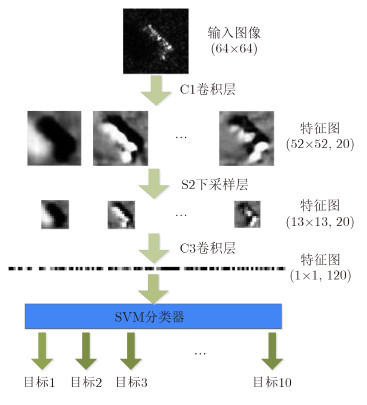﻿ 基于卷积神经网络的SAR图像目标识别研究
«上一篇文章快速检索 高级检索

 雷达学报2016, Vol. 5Issue (3): 320-325  DOI: 10.12000/JR160370

### 引用本文 [复制中英文]

[复制中文]
Tian Zhuangzhuang, Zhan Ronghui, Hu Jiemin, Zhang Jun, et al. SAR ATR Based on Convolutional Neural Network[J]. Journal of Radars, 2016, 5(3): 320-325. DOI: 10.12000/JR16037.
[复制英文]

### 文章历史, , ,
(国防科学技术大学自动目标识别重点实验室 长沙 410073)

SAR ATR Based on Convolutional Neural Network, , ,
ATR Key Laboratory, National University of Defense Technology, Changsha 410073, China
Foundation Item: The National Natural Science Foundation of China (61471370)
Abstract: This study presents a new method of Synthetic Aperture Radar (SAR) image target recognition based on a convolutional neural network. First, we introduce a class separability measure into the cost function to improve this network's ability to distinguish between categories. Then, we extract SAR image features using the improved convolutional neural network and classify these features using a support vector machine. Experimental results using moving and stationary target acquisition and recognition SAR datasets prove the validity of this method.
Key words: Synthetic Aperture Radar (SAR)     Automatic Target Recognition (ATR)     Convolutional Neural Network (CNN)     Support Vector Machine (SVM)     Back Propagation (BP)

1 引言

CNN主要有以下特点：采用原始图像作为网络输入，避免了复杂的特征提取过程；局部感受域获取的特征与图像的平移、缩放和旋转无关；权值共享结构降低了网络模型的复杂度；下采样利用局部相关性原理，在有效减少数据处理量的同时保留了结构信息。CNN作为一种特征学习方法，通过非线性的简单模型将原始数据转化为更为抽象的表达，在特征学习过程中不需要过多的人工参与

2 卷积神经网络

 $\left. \begin{array}{l} {{u}^l} = {{W}^l}{{x}^{l - 1}} + {{b}^l}\\ {{x}^l} = f\left( {{{u}^l}} \right) \end{array} \right\}$ (1)

 ${E_0} = \frac{1}{2}\sum\limits_{k = 1}^B {{{\left( {t_k^n - y_k^n} \right)}^2}} = \frac{1}{2}\left\| {{{t}^n} - {{y}^n}} \right\|_2^2$ (2)

 ${{\delta }^L} = f'({{u}^L}) \circ ({{y}^n} - {{t}^n})$ (3)

 ${{\delta }^l} = {{W}^{l + 1}}{{\delta }^{l + 1}} \circ f'({{u}^l})$ (4)

 $\left. \begin{array}{l} \frac{{\partial {E_0}}}{{\partial {{W}^l}}} = \frac{{\partial {E_0}}}{{\partial {{u}^l}}} \circ \frac{{\partial {{u}^l}}}{{\partial {{W}^l}}} = {{\delta }^l} \circ {{x}^{l - 1}}\\ \frac{{\partial {E_0}}}{{\partial {{b}^l}}} = \frac{{\partial {E_0}}}{{\partial {{u}^l}}} \circ \frac{{\partial {{u}^l}}}{{\partial {{b}^l}}} = {{\delta }^l} \end{array} \right\}$ (5)

 $\left. \begin{array}{l} \Delta\! {{W}^l} = - \eta \frac{{\partial {E_0}}}{{\partial {{W}^l}}}\\ \Delta \!{{b}^l} = - \eta \frac{{\partial {E_0}}}{{\partial {{b}^l}}} \end{array} \right\}$ (6)

3 卷积神经网络的改进 3.1 引入类别可分性度量

 ${E_1} = \frac{1}{2}\left\| {{y}_c^n - {{M}_c}} \right\|_2^2 \quad \quad \$ (7)
 ${E_2} = \frac{1}{2}\sum\limits_{c' = 1}^B {\left\| {{{M}_c} - {{M}_{c'}}} \right\|_2^2}$ (8)

 $E = {E_0} + \alpha {E_1} - \beta {E_2}$ (9)

 $\delta _{1}^{L}=\frac{\partial {{E}_{1}}}{\partial {{u}^{L}}}=\frac{\partial }{\partial {{u}^{L}}}\frac{1}{2}\left\| y_{c}^{n}-{{M}_{c}} \right\|_{2}^{2}=\left( 1-\frac{1}{{{n}_{c}}} \right){f}'\left( {{u}^{L}} \right){}^\circ (y_{c}^{n}-{{M}_{c}})$ (10)

 \begin{align} & \delta _{2}^{L}=\frac{\partial {{E}_{2}}}{\partial {{u}^{L}}}=\frac{\partial }{\partial {{u}^{L}}}\frac{1}{2}\sum\limits_{{c}'=1}^{B}{\left\| {{M}_{c}}-{{M}_{{{c}'}}} \right\|_{2}^{2}}= \\ & \frac{1}{{{n}_{c}}}{f}'({{u}^{L}}){}^\circ \sum\limits_{{c}'=1}^{B}{\left( {{M}_{c}}-{{M}_{{{c}'}}} \right)} \\ \end{align} (11)

 \begin{align} & {{\mathbf{\delta }}^{\prime }}={{\delta }^{L}}+\alpha \delta _{1}^{L}-\beta \delta _{2}^{L}= \\ & {f}'({{u}^{L}}){}^\circ ({{y}^{n}}-{{t}^{n}})+\alpha \left( 1-\frac{1}{{{n}_{c}}} \right)\cdot \\ & {f}'({{u}^{L}}){}^\circ (y_{c}^{n}-{{M}_{c}}) \\ & -\beta \frac{1}{{{n}_{c}}}{f}'({{u}^{L}}){}^\circ \sum\limits_{{c}'=1}^{B}{\left( {{M}_{c}}-{{M}_{{{c}'}}} \right)} \\ \end{align} (12)

3.2 支持向量机的使用

4 实验结果 4.1 实验数据集

4.2 实验模型图 1 本文卷积神经网络结构示意图 Fig. 1 CNN structural plan of the method
4.3 3 类目标的识别结果与分析表 1 3类MSTAR目标识别结果 Tab. 1 The recognition results of three types of MSTAR targets表 2 与其他方法的效果对比 Tab. 2 The recognition performance compare with >other methods
4.4 10类目标的识别结果与分析表 3 10类MSTAR目标识别结果 Tab. 3 The recognition results of ten types of MSTAR targets
5 结论

  Ross T D, Worrell S W, Velten V J, et al.. Standard SAR ATR evaluation experiments using the MSTAR public release data set[C]. Aerospace/Defense Sensing and Controls, International Society for Optics and Photonics, 1998: 566-573.(1)  Tao W, Xi C, Xiangwei R, et al.. Study on SAR target recognition based on support vector machine[C]. 2009 2nd Asian-Pacific Conference on Synthetic Aperture Radar, 2009: 856-859.(1)  王璐, 张帆, 李伟, 等. 基于 Gabor 滤波器和局部纹理特征提取的 SAR 目标识别算法[J]. 雷达学报, 2015, 4(6): 658-665. DOI: 10.12000/JR15076. Wang Lu, Zhang Fan, Li Wei, et al.. A method of SAR target recognition based on Gabor filter and local texture feature extraction[J]. Journal of Radars, 2015, 4(6); 658-665. DOI: 10.12000/JR15076.(1)  齐会娇, 王英华, 丁军, 等. 基于多信息字典学习及稀疏表示的SAR目标识别[J]. 系统工程与电子技术, 2015, 37(6): 1280-1287. Qi Huijiao, Wang Yinghua, Ding Jun, et al.. SAR target recognition based on multi-information dictionary learning and sparse representation[J]. System Engineering and Electronics, 2015, 37(6): 1280-1287.(1)  Hinton G E and Salakhutdinov R R. Reducing the dimensionality of data with neural networks[J]. Science, 2006, 313(5786): 504-507.(1)  Hinton G E, Osindero S, and Teh Y W. A fast learning algorithm for deep belief nets[J]. Neural Computation, 2006, 18(7): 1527-1554.(1)  Vincent P, Larochelle H, Lajoie I, et al.. Stacked denoisingautoencoders: learning useful representations in a deep network with a local denoisingcriterion[J]. The Journal of Machine Learning Research, 2010, 11: 3371-3408.(1)  Lecun Y, Bottou L, Bengio Y, et al.. Gradient-based learning applied to document recognition[J]. Proceedings of the IEEE, 1998, 86(11): 2278-2324.(1)  Ma Y, He J, Wu L, et al.. An effective face verification algorithm to fuse complete features in convolutional neural network[C]. MultiMedia Modeling. Springer International Publishing, 2016: 39-46.(1)  Ijjina E P and Mohan C K. Human action recognition based on motion capture information using fuzzy convolution neural networks[C]. 2015 Eighth International Conference on Advances in Pattern Recognition (ICAPR), Kalkata, 2015: 1-6.(1)  Ciompi F, de Hoop B, van Riel S J, et al.. Automatic classification of pulmonary peri-fissural nodules in computed tomography using an ensemble of 2D views and a convolutional neural network out-of-the-box[J]. Medical Image Analysis, 2015, 26(1): 195-202.(1)  尹宝才, 王文通, 王立春. 深度学习研究综述[J]. 北京工业大学学报, 2015, 41(1): 48-59. Yin Bao-cai, Wang Wen-tong, and Wang Li-chun. Review of deep learning[J]. Journal of Beijing University of Technology, 2015, 41(1): 48-59.(1)  Lecun Y, Bengio Y, and Hinton G. Deep Learning[J]. Nature, 2015, 521(7553): 436-444.(1)  Rumelhart D E, Hinton G E, and Williams R J. Learning representations by back-propagating errors[J]. Nature, 1986, 323(6088): 533-536.(1)  孙艳丰, 齐光磊, 胡永利, 等. 基于改进 Fisher 准则的深度卷积神经网络识别算法[J]. 北京工业大学学报, 2015, 41(6): 835-841. Sun Yanfeng, Qi Guanglei, Hu Yongli, et al.. Deep convolution neural network recognition algorithm based on improved fisher criterion[J]. Journal of Beijing University of Technology, 2015, 41(6): 835-841.(1)  Cortes C and Vapnik V. Support-vector networks[J]. Machine Learning, 1995, 20(3): 273-297.(1)  孙即祥, 等. 现代模式识别[M]. 北京: 高等教育出版社, 2008: 624-625. Sun Jixiang, et al.. Pattern Recognition[M]. Beijing: Higher Education Press, 2008: 624-625.(1)  Glorot X and Bengio Y. Understanding the difficulty of training deep feedforward neural networks[J]. Journal of Machine Learning Research, 2010, 9: 249-256. (1)